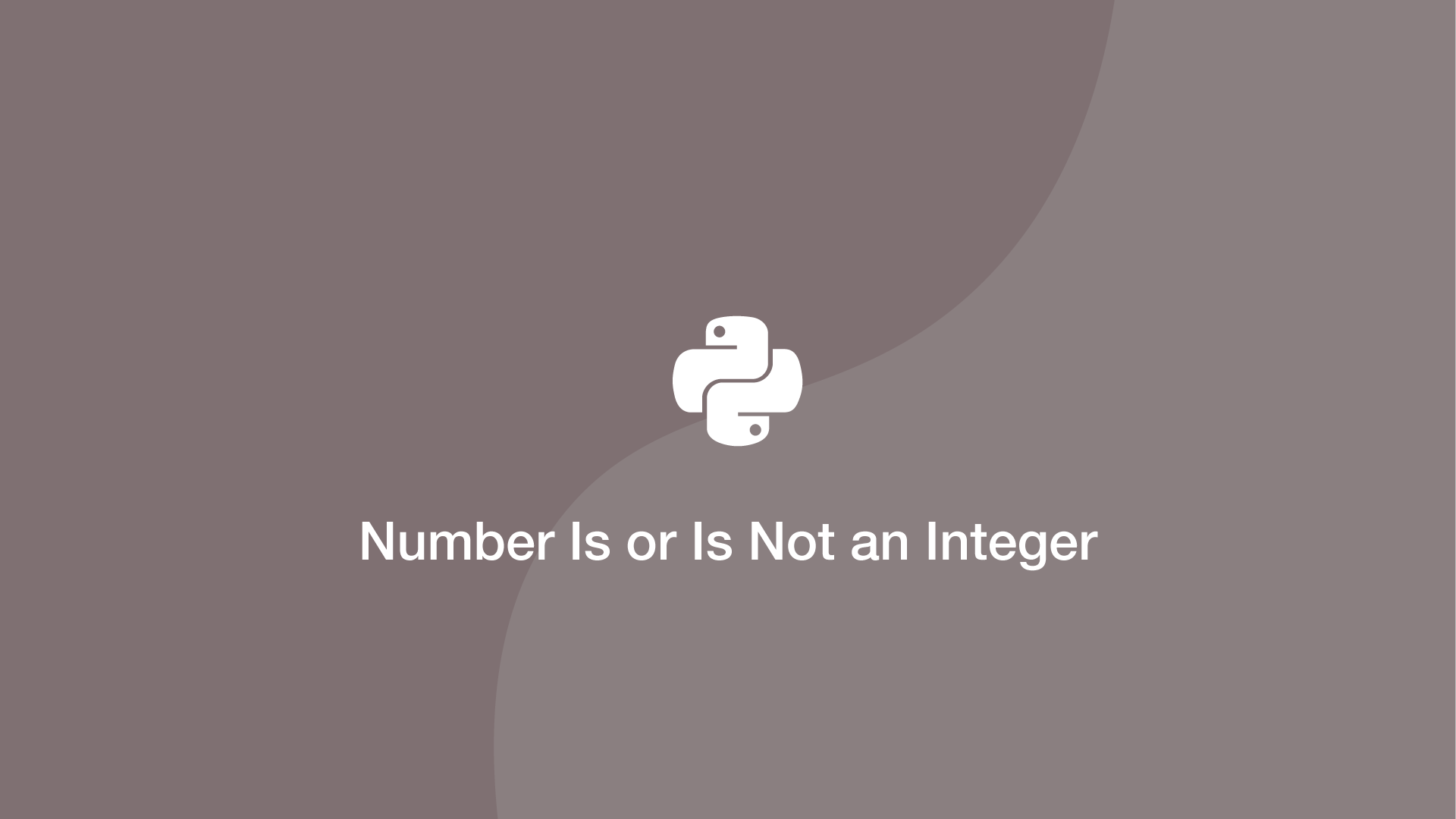# How to Check if a Number Is or Is Not an Integer in PythonIn this tutorial, we will learn how to check if a number with the type of `float` is or is not an integer (whole number) in Python.

## Check Number is an Integer with the Python is_integer() Function

Let's say we have a whole number of the datatype float and need to know that it is indeed a whole number. We can evaluate this with the built-in Python `is_integer()` function with an if block.

``````number = float(22)

if number.is_integer():
print(f'{number} is an integer')
else:
print(f'{number} is not an integer')
``````
``````22.0 is an integer
``````

## Check a Float is not an Integer in Python

If we need to know if a floating-point number is not an integer, we can use the is_integer() function with an `is not True` statement.

``````number = float(22.1)

if number.is_integer() is not True:
print('Not an integer')
``````
``````Not an integer
``````

#### Related Tutorials### How to Convert a String to a Number in Python

October 01, 2020### How to Use Argparse in Python

September 25, 2020### How to Hash Passwords in PHP

July 08, 2020### How to Round Numbers Up and Down in Python

September 28, 2020### How to use Sets in Python

September 15, 2020### How to Compare Strings in Python

September 20, 2020